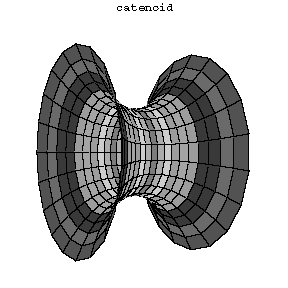Catenoid

(diff) ← Older revision | Latest revision (diff) | Newer revision → (diff)
The surface formed by the revolution of a catenary $y=c\cosh x/c$ about the $x$-axis, where $c$ is a positive constant. The parametric equations for the catenoid are then $x = v \quad y = c \cosh \frac{v}{c} \sin u \quad z = c \cosh \frac{v}{c} \cos u\, .$ The catenoid is a minimal surface and it is the form realized by a soap film "stretched" over two wire discs the planes of which are perpendicular to the line joining their centres (see Fig. 1). The catenoid is a member of the one-parameter family of surfaces of revolution of the curves $y = a \cosh x/b$, which are sometimes also called catenoids. However only for the special choice of parameters $a = b$ the corresponding surface is a minimal surface and usually the word catenoid is used only for this particular case.